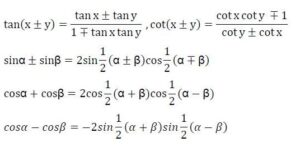# Revised CBSE Syllabus 2021 for Class 11 Mathematics

### Revised CBSE Syllabus 2021 for Class 11 Mathematics

#### Max Marks: 80

 No. Units No. of Periods Marks I. Sets and Functions 43 23 II. Algebra 41 30 III. Coordinate Geometry 33 10 IV. Calculus 30 07 V. Statistics and Probability 21 10 Total 168 80 Internal Assessment 20

*No chapter/unit-wise weightage. Care to be taken to cover all the chapters.

### Unit-I: Sets and Functions

#### 1. Sets (14) Periods

Sets and their representations. Empty set. Finite and Infinite sets. Equal sets. Subsets. Subsets of a set of real numbers especially intervals (with notations). Power set. Universal set. Venn diagrams. Union and Intersection of sets.

#### 2. Relations & Functions (15) Periods

Ordered pairs. Cartesian product of sets. Number of elements in the Cartesian product of two finite sets. Cartesian product of the set of reals with itself ( R x R only).Definition of relation, pictorial diagrams, domain, co-domain and range of a relation. Function as a special type of relation. Pictorial representation of a function, domain, co-domain and range of a function. Real valued functions, domain and range of these functions, constant, identity, polynomial, rational, modulus, signum, exponential, logarithmic and greatest integer functions, with their graphs.

#### 3. Trigonometric Functions (14) Periods

Positive and negative angles. Measuring angles in radians and in degrees and conversion from one measure to another. Definition of trigonometric functions with the help of unit circle. Truth of the identity sin2x + cos2x = 1, for all x. Signs of trigonometric functions. Domain and range of trigonometric functions and their graphs. Expressing sin (x±y) and cos (x±y) in terms of sinx, siny, cosx & cosy and their simple applications. Deducing identities like the following:Identities related to sin2x, cos2x, tan2 x, sin3x, cos3x and tan3x.

### Unit-II: Algebra

#### 1. Complex Numbers and Quadratic Equations (10) Periods

Need for complex numbers, especially√−1, to be motivated by inability to solve some of the quardratic equations. Algebraic properties of complex numbers. Argand plane. Statement of Fundamental Theorem of Algebra, solution of quadratic equations (with real coefficients) in the complex number system.

#### 2. Linear Inequalities (15) Periods

Linear inequalities. Algebraic solutions of linear inequalities in one variable and their representation on the number line. Graphical solution of linear inequalities in two variables. Graphical method of finding a solution of system of linear inequalities in two variables.

#### 3. Permutations and Combinations (8) Periods

Fundamental principle of counting. Factorial n. (n!) Permutations and combinations, formula for nPr and nCr, simple applications.

### 4. Sequence and Series (8) Periods

Sequence and Series. Arithmetic Progression (A. P.). Arithmetic Mean (A.M.) Geometric Progression (G.P.), general term of a G.P., sum of n terms of a G.P., infinite G.P. and its sum, geometric mean (G.M.), relation between A.M. and G.M.

### Unit-III: Coordinate Geometry

#### 1. Straight Lines (8) Periods

Brief recall of two dimensional geometry from earlier classes. Slope of a line and angle between two lines. Various forms of equations of a line: parallel to axis, point -slope form, slope-intercept form, two-point form, intercept form and normal form. General equation of a line. Distance of a point from a line.

#### 2. Conic Sections (15) Periods

Sections of a cone: circles, ellipse, parabola, hyperbola. Standard equations and simple properties of parabola, ellipse and hyperbola. Standard equation of a circle.

#### 3. Introduction to Three-dimensional Geometry (10) Periods

Coordinate axes and coordinate planes in three dimensions. Coordinates of a point. Distance between two points and section formula.

### Unit-IV: Calculus

#### 1. Limits and Derivatives (30) Periods

Derivative introduced as rate of change both as that of distance function and geometrically. Intuitive idea of limit. Limits of polynomials and rational functions trigonometric, exponential and logarithmic functions .Definition of derivative relate it to scope of tangent of the curve, derivative of sum, difference, product and quotient of functions. Derivatives of polynomial and trigonometric functions.

### Unit-V: Statistics and Probability

#### 1. Statistics (11) Periods

Measures of Dispersion: Range, Mean deviation, variance and standard deviation of ungrouped/grouped data.

#### 2. Probability (10) Periods

Random experiments; outcomes, sample spaces (set representation). Events; occurrence of events, ‘not’, ‘and’ and ‘or’ events, exhaustive events, mutually exclusive events, Probability of an event, probability of ‘not’, ‘and’ and ‘or’ events.

### MATHEMATICS QUESTION PAPER DESIGN

CLASS – XI (2020-21)

Time : 3 Hours

Max. Marks: 80

 S.No. Typology of Questions Total Marks % Weight age 1 Remembering: Exhibit memory of previously learned material by recalling facts, terms, basic concepts, and answers. Understanding: Demonstrate understanding of facts and ideas by organizing, comparing, translating, interpreting, giving descriptions, and stating main ideas 44 55 2 Applying: Solve problems to new situations by applying acquired knowledge, facts, techniques and rules in a different way. 20 25 3 Analysing : Examine and break information into parts by identifying motives or causes. Make inferences and find evidence to support generalizations Evaluating: Present and defend opinions by making judgments about information, validity of ideas, or quality of work based on a set of criteria.  Creating: Compile information together in a different way by combining elements in a new pattern or proposing alternative solutions 16 20 Total 80 100
1. No chapter wise weightage. Care to be taken to cover all the chapters
2. Suitable internal variations may be made for generating various templates keeping the overall weightage to different form of questions and typology of questions same.

### Choice(s):

There will be no overall choice in the question paper. However, 33% internal choices will be given in all the sections

 INTERNAL ASSESSMENT                                                                                      20 MARKS Periodic Tests ( Best 2 out of 3 tests conducted)                                         10 Marks Mathematics Activities                                                                                            10 Marks

Revised CBSE Syllabus 2021 for Class 11 Computer Science